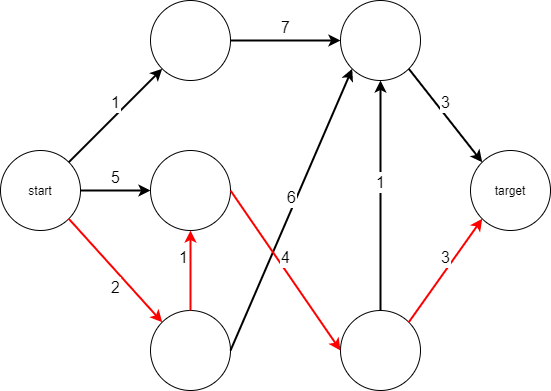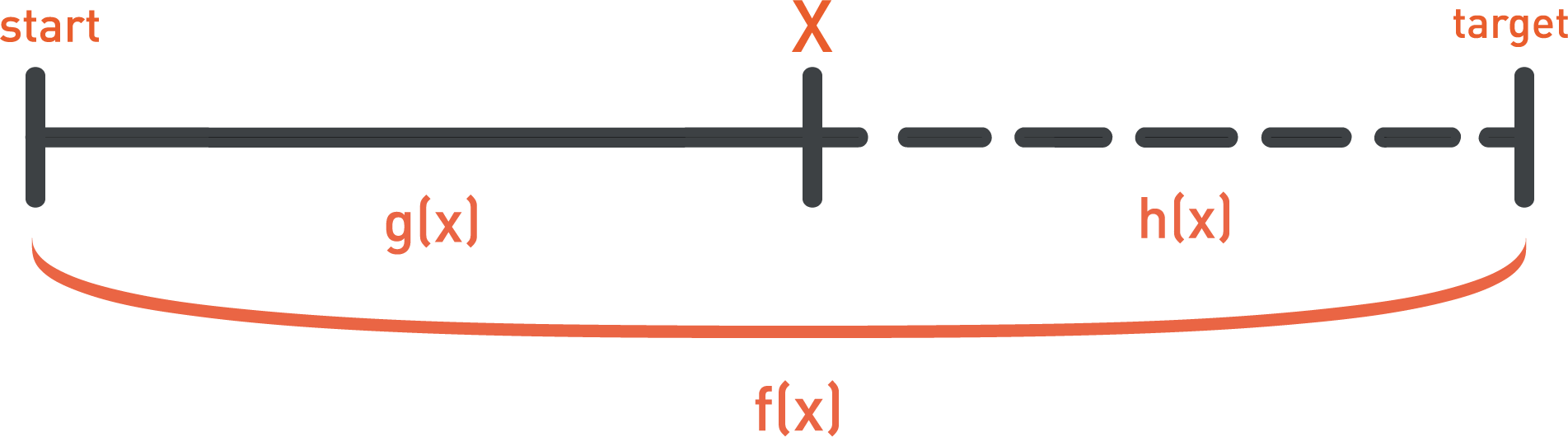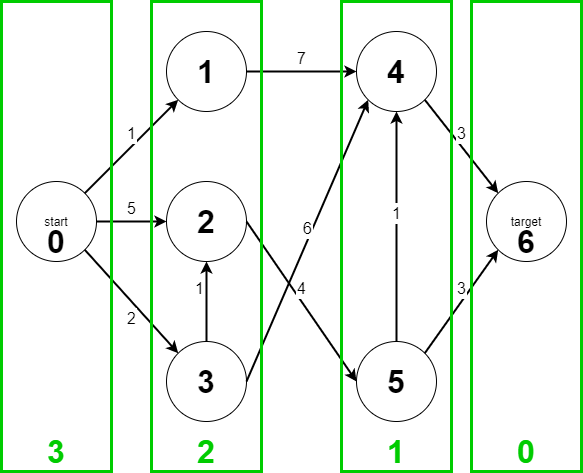Graphs in Java - A* Algorithm - Stack Abuse

# Graphs in Java - A* Algorithm### Introduction

A* is a heuristic path searching graph algorithm. This means that given a weighed graph, it outputs the shortest path between two given nodes.

The algorithm is guaranteed to terminate for finite graphs with non-negative edge weights. Additionally, if you manage to ensure certain properties when designing your heuristic it will also always return an almost-optimal solution in a pretty efficient manner.

A heuristic is a method which is constructed to guide us to the optimal solution most of the time, which means we trade in some accuracy for a lot of speed (if the heuristic is well constructed).In this article, we'll go over:

• Some characteristics that we aim to have in our heuristic search algorithms in general.
• Show a logical progression from a greedy search to A*.
• Go through the aforementioned conditions which enable A* to solve our problem optimally and efficiently.

Table of Contents:

### Graph Search Characteristics

We'll begin by outlining some things that we tend to want to accomplish with our algorithm.

The following are all very important metrics that separate A* from other similar algorithms and should thus be understood thoroughly if we want to meaningfully apply it in practice:

1. Completeness - is a property of an algorithm that ensures an algorithm will terminate with a solution if a solution exists.
2. Optimality - is a property that guarantees that our algorithm's solution will be the best available solution based on the criteria we set as our goal.
3. Time and memory complexity - measures the efficiency of our algorithm's resource usage and thus its practical applicability.

### Shortfalls of Other Algorithms

When faced with the problem of finding the shortest path in a graph in a reasonable amount of time, many of us would be tempted to sacrifice optimality and go for the greedy solution - always picking the edge with the lowest weight - going along the stream with the least resistance.

An observant reader might notice that by doing that, we've also sacrificed completeness - greedy search can sometimes get stuck in infinite loops. We can do better than that.

If you've thought of Dijkstra's algorithm, points for you! That's a great algorithm for finding the shortest path and is also pretty efficient. It does the job even for huge scale calculations, such as routing across the entirety of the Internet. It's also both complete and optimal.

So job's done, right?

Not so quickly.

While Dijkstra may be the best possible solution for some real-world problems, it can spend a lot of time checking alternative paths, especially in a dense graph with many nodes. In fact, Dijkstra evaluates every node in the graph. Even the ones behind it, going away from the goal. If the goal was right in front of the current node, it'd still evaluate the nodes on the opposite side of the graph, even though it could just evaluate the intermediary nodes between itself and the goal.

It's just like taking a look at the entire map of the city on every step you make towards a coffee shop, instead of directing your search in the general direction of the shop.

If we could somehow guide the general direction it goes in, towards the target node, we could skip a lot of unnecessary work.

Let's say we're able to roughly guess the distance between two nodes. Perhaps we're trying to calculate a travel path by road between two points on Earth. We could say that the straight airplane travel distance is a rough estimation of how far apart they are. What if we used this estimation to pick the next node instead of using the edge weight?

That approach is called best-first search and will often increase our efficiency, but we will often end up with a suboptimal solution.

That leads us to how A* manages to solve all of these problems.

Note: Some refer to A* as the informed Dijkstra.

### The A* Algorithm in Java

Starting conditions:

• We have a starting node (called start) and a target node (called target).
• We have a weighted directed graph of n nodes.

The goal:

• Find the shortest path from start to finish

#### Cost Function - f(n)

We want to determine which node to move into at every step. To do that, we'll design a mathematical function f(n) which will measure how good of a candidate a node is for being included in our shortest path.

This is the cost function, and we'll want to minimize it to produce an optimal outcome.

The cost function is the sum of a move function and a heuristic function.

#### Move Function - g(n)

Because we're at node n, we know the cost it took us to get there from the start node. We'll call that move function - g(n).

If we say that f(n)=g(n) we'll create Dijkstra's algorithm. At each step, we'd be picking the node with the lowest cost to get to from start - the node with the smallest value for g(n). This means our function is lacking a "guiding component" so to speak.

#### Heuristic Function - h(n)

We'll call this guiding component a heuristic and label it h(n). We'll use this component to estimate how close the node we're looking at is to the target.

This estimation is the heart and soul of A* and it will make or break any particular implementation of it, but theoretically speaking you can use any function you'd like. If we knew the exact distance in terms of nodes, we'd already have the optimal solution.

Though, if we know the position of the target node, we can for example, calculate the Euclidean Distance between the target node and our current node. The shorter it is, the closer we are to the target node - roughly.

Note: You'll just get better results if you carefully craft your heuristic.

#### Calculating A* Moves

So the final formula we get is f(n)=g(n)+h(n). We start from the start node, add it to a list of open nodes. We evaluate all of the open nodes' neighbors and add them to the list of open nodes. We pick the one with the lowest value for f(n) and if it's not the target we repeat the process.

The fewer steps we take from the starting point combined with how close we get to the goal makes the value of f(n) lower if we're going with the shortest path to the goal. Walking away from the goal, and making more steps than needed to get there increases the f(n) function.

If you're confused a bit with the difference between g(n) and h(n), look at it like this:

• g is something we can (and do) calculate at any given step, and it's the distance between start and n.
• h is something we don't know, and need to estimate - the distance from n to the target node.
• f is the sum of the two#### A* Pseudocode

We maintain two lists of nodes, an open list and a closed list.

The open list contains nodes that we've encountered, but haven't analyzed yet. Initially, it only contains the starting node.

The closed list contains nodes whose all neighbors have been added to the open list. Closed nodes have their shortest path calculated and their adjacent nodes "scheduled" for analysis by being added to the open list.

Closed nodes can become open again if we encounter them through a different path and that path is more optimal than the one we previously used to reach them.

We go through open nodes, open their neighbors, calculate their f and g and then close them again.

## Git Essentials

Check out this hands-on, practical guide to learning Git, with best-practices and industry-accepted standards. Stop Googling Git commands and actually learn it!

Usually you'd need to calculate h once, the first time you encounter a node. You don't have to recalculate it multiple times because it's fixed. We've omitted that in this code, assuming the heuristic is calculated in advance, but you can add it depending on your application:


make an empty list C of closed nodes
make a list O of open nodes and their respective f values containing the start node
while O isn't empty:
pick a node n from O with the best value for f
if n is target:
return solution
for every m which is a neighbor of n:
if (m is not in C) and (m is not in O):
add m to O, set n as m's parent
calculate g(m) and f(m) and save them
else:
if f(m) from last iteration is better than g(m) from this iteration:
set n as m's parent
update g(m) and f(m)
if m is in C:
move m to O
move n from O to C

return that there's no solution



#### A* Implementation in Java

We'll implement an algorithm for the graph shown in the beginning of the article. Our heuristic will treat each "layer" as a step towards the target node. The numbers inside of the nodes are their IDs, which we'll use to print out the resulting path:Note: This is not a good heuristic in practice.

Each problem will have its own fitting heuristic, because a graph can be drawn in many ways - nodes can appear closer or further from the target than they actually are when considering the weight of edges

We've gone with this approach for illustrative purposes and in the next section, we'll delve deeper into how to make a useful heuristic in practice.

Let's make a Node class to represent a node in our graph:

public class Node implements Comparable<Node> {
// Id for readability of result purposes
private static int idCounter = 0;
public int id;

// Parent in the path
public Node parent = null;

public List<Edge> neighbors;

// Evaluation functions
public double f = Double.MAX_VALUE;
public double g = Double.MAX_VALUE;
// Hardcoded heuristic
public double h;

Node(double h){
this.h = h;
this.id = idCounter++;
this.neighbors = new ArrayList<>();
}

@Override
public int compareTo(Node n) {
return Double.compare(this.f, n.f);
}

public static class Edge {
Edge(int weight, Node node){
this.weight = weight;
this.node = node;
}

public int weight;
public Node node;
}

public void addBranch(int weight, Node node){
Edge newEdge = new Edge(weight, node);
neighbors.add(newEdge);
}

public double calculateHeuristic(Node target){
return this.h;
}
}


And here's the algorithm itself:

public static Node aStar(Node start, Node target){
PriorityQueue<Node> closedList = new PriorityQueue<>();
PriorityQueue<Node> openList = new PriorityQueue<>();

start.f = start.g + start.calculateHeuristic(target);
openList.add(start);

while(!openList.isEmpty()){
Node n = openList.peek();
if(n == target){
return n;
}

for(Node.Edge edge : n.neighbors){
Node m = edge.node;
double totalWeight = n.g + edge.weight;

if(!openList.contains(m) && !closedList.contains(m)){
m.parent = n;
m.g = totalWeight;
m.f = m.g + m.calculateHeuristic(target);
openList.add(m);
} else {
if(totalWeight < m.g){
m.parent = n;
m.g = totalWeight;
m.f = m.g + m.calculateHeuristic(target);

if(closedList.contains(m)){
closedList.remove(m);
openList.add(m);
}
}
}
}

openList.remove(n);
closedList.add(n);
}
return null;
}

public static void printPath(Node target){
Node n = target;

if(n==null)
return;

List<Integer> ids = new ArrayList<>();

while(n.parent != null){
ids.add(n.id);
n = n.parent;
}
ids.add(n.id);
Collections.reverse(ids);

for(int id : ids){
System.out.print(id + " ");
}
System.out.println("");
}


And now, let's construct a graph and call this method:

public static void main(String[] args) {
Node head = new Node(3);
head.g = 0;

Node n1 = new Node(2);
Node n2 = new Node(2);
Node n3 = new Node(2);

head.addBranch(1, n1);
head.addBranch(5, n2);
head.addBranch(2, n3);
n3.addBranch(1, n2);

Node n4 = new Node(1);
Node n5 = new Node(1);
Node target = new Node(0);

n1.addBranch(7, n4);
n2.addBranch(4, n5);
n3.addBranch(6, n4);

n4.addBranch(3, target);
n5.addBranch(1, n4);
n5.addBranch(3, target);

Node res = aStar(head, target);
printPath(res);
}


When we run this, we'll get the result printed out:

0 3 2 5 6### Creating a Good Heuristic Function

#### Admissibility and Consistency

The performance of A* hinges on using a good heuristic. The algorithm itself can have some very useful properties if we ensure that the heuristic follows certain rules. Let's take a look.

Function h(n) is admissible if it never overestimates the real distance between the current node and the target. Meaning that the following inequality is true for every node n:

$$h(n)\leq h\ ⃰(n)$$

Where h ⃰ is the ideal heuristic, accurately measuring the shortest path.

If h is admissible, A* will always return the optimal path.

If h is not admissible, but it doesn't overestimate the real distance by more than some value d, then the length of the path found by A* won't differ from the optimal path by more than d.

Function h(n) is consistent if it evaluates to 0 for the target node and if for every two neighboring nodes it is true that:

$$c(n,m)+h(m)\geq h(n)$$

Where c(n,m) is the weight of the edge (n,m).

Theorem: If a heuristic function is consistent, then it is also admissible.

The proof of this theorem is done by complete induction.

#### Complexity

Barring special cases, complexity of A* can be approximated based on the number of neighbors of every node and the length of the shortest path. Let's say that every node has at most b neighbors and the shortest path is of distance d. The complexity of A* is then:

$$O(b^d)$$

Exponential complexity would be no better than brute force, so this may seem bad. The thing is, we can lower this to polynomial complexity if our heuristic satisfies the following equation:

$$|h(x)-h\ ⃰(x)| \leq O(\log h\ ⃰(x))$$

A* is also optimally efficient, meaning that it has been proven that no complete algorithm is more efficient than A* for solving the same problem.

### Example - 2D Terrain With Obstacles

Let's say we have a 2D grid with obstacles. Each square corresponds to one node and we can move like a king in chess - one square horizontally, vertically, or diagonally. We want to find the shortest path from start to target.#### Representation

In this case, we can represent our graph as a matrix of nodes, rather than using adjacency lists. Each node can have an indicator of whether it's walkable or an obstacle. We can use matrix indices to figure out adjacent nodes as well as to use them like they're coordinates when calculating our heuristic distances.

#### Heuristic

Your first thought might be using Euclidean distance. However, in large problems, this should be avoided as calculating the square root often can cause inefficiency. It's a good metric if nothing else fits the problem, but if you can get away with using a simplified distance you should try to.

A second idea might be Manhattan distance (also called taxicab or city-block distance). Manhattan distance the sum of horizontal and vertical differences:

$$D_{Manhattan}(p,q)=|q_x-p_x|+|q_y-p_y|$$

However, this metric is not admissible because it often overestimates the distance. Imagine a grid with no obstacles and start and target positioned diagonally. Manhattan would always overestimate this case.

A good choice, in this case, is so-called Chebyshev distance:

$$D_{Chebyshev}(p,q)=max(|q_x-p_x|,|q_y-p_y|)$$

This metric is admissible and thus guarantees an optimal solution. It's also quick to calculate, so it doesn't put a strain on resources in each iteration.

### Conclusion

We've taken a look at A* search algorithm and its properties. We've learned how it works and why it's very good in practice, provided that we can ensure certain properties of a heuristic guiding it.

Applying this to real problems takes practice and experience, but this article should have given the reader a good foundation to start them off.

Last Updated: March 22nd, 2021

## Improve your dev skills!

Get tutorials, guides, and dev jobs in your inbox.

No spam ever. Unsubscribe at any time. Read our Privacy Policy.

# Prepping for an interview?

• Improve your skills by solving one coding problem every day
• Get the solutions the next morning via email
• Practice on actual problems asked by top companies, like:

# Git Essentials

Check out this hands-on, practical guide to learning Git, with best-practices and industry-accepted standards. Stop Googling Git commands and actually learn it!

© 2013-2021 Stack Abuse. All rights reserved.